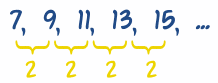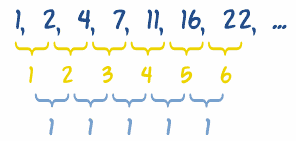# Sequences - Finding a Rule

To find a missing number in a Sequence, first we must have a Rule

## Sequence

A Sequence is a set of things (usually numbers) that are in order.Each number in the sequence is called a term (or sometimes "element" or "member"), read Sequences and Series for a more in-depth discussion.

## Finding Missing Numbers

To find a missing number, first find a Rule behind the Sequence.

Sometimes we can just look at the numbers and see a pattern:

### Example: 1, 4, 9, 16, ?

Answer: they are Squares (12=1, 22=4, 32=9, 42=16, ...)

Rule: xn = n2

Sequence: 1, 4, 9, 16, 25, 36, 49, ...

Did you see how we wrote that rule using "x" and "n" ?

xn means "term number n", so term 3 is written x3

And we can calculate term 3 using:

x3 = 32 = 9

We can use a Rule to find any term. For example, the 25th term can be found by "plugging in" 25 wherever n is.

x25 = 252 = 625

### Example: 3, 5, 8, 13, 21, ?

After 3 and 5 all the rest are the sum of the two numbers before,

That is 3 + 5 = 8, 5 + 8 = 13 etc, which is part of the Fibonacci Sequence:

3, 5, 8, 13, 21, 34, 55, 89, ...

Which has this Rule:

Rule: xn = xn-1 + xn-2

Now what does xn-1 mean? It means "the previous term" as term number n-1 is 1 less than term number n.

And xn-2 means the term before that one.

Let's try that Rule for the 6th term:

x6 = x6-1 + x6-2

x6 = x5 + x4

So term 6 equals term 5 plus term 4. We already know term 5 is 21 and term 4 is 13, so:

x6 = 21 + 13 = 34

## Many Rules

One of the troubles with finding "the next number" in a sequence is that mathematics is so powerful we can find more than one Rule that works.

### What is the next number in the sequence 1, 2, 4, 7, ?

Here are three solutions (there can be more!):

So, 1+1=2, 2+2=4, 4+3=7, 7+4=11, etc...

Rule: xn = n(n-1)/2 + 1

Sequence: 1, 2, 4, 7, 11, 16, 22, ...

(That rule looks a bit complicated, but it works)

Solution 2: After 1 and 2, add the two previous numbers, plus 1:

Rule: xn = xn-1 + xn-2 + 1

Sequence: 1, 2, 4, 7, 12, 20, 33, ...

Solution 3: After 1, 2 and 4, add the three previous numbers

Rule: xn = xn-1 + xn-2 + xn-3

Sequence: 1, 2, 4, 7, 13, 24, 44, ...

So, we have three perfectly reasonable solutions, and they create totally different sequences.

Which is right? They are all right.

And there are other solutions ...... it may be a list of the winners' numbers ... so the next number could be ... anything!

## Simplest Rule

When in doubt choose the simplest rule that makes sense, but also mention that there are other solutions.

## Finding Differences

Sometimes it helps to find the differences between each pair of numbers ... this can often reveal an underlying pattern.

Here is a simple case:The differences are always 2, so we can guess that "2n" is part of the answer.

Let us try 2n:

n: 1 2 3 4 5
Terms (xn): 7 9 11 13 15
2n: 2 4 6 8 10
Wrong by: 5 5 5 5 5

The last row shows that we are always wrong by 5, so just add 5 and we are done:

Rule: xn = 2n + 5

OK, we could have worked out "2n+5" by just playing around with the numbers a bit, but we want a systematic way to do it, for when the sequences get more complicated.

## Second Differences

In the sequence {1, 2, 4, 7, 11, 16, 22, ...} we need to find the differences ...

... and then find the differences of those (called second differences), like this:The second differences in this case are 1.

With second differences we multiply by n22

In our case the difference is 1, so let us try just n22:

n: 1 2 3 4 5
Terms (xn): 1 2 4 7 11

n22: 0.5 2 4.5 8 12.5
Wrong by: 0.5 0 -0.5 -1 -1.5

We are close, but seem to be drifting by 0.5, so let us try: n22n2

 n22 − n2 Wrong by: 0 1 3 6 10 1 1 1 1 1

Wrong by 1 now, so let us add 1:

 n22 − n2 + 1 Wrong by: 1 2 4 7 11 0 0 0 0 0

We did it!

The formula n22n2 + 1 can be simplified to n(n-1)/2 + 1

So by "trial-and-error" we discovered a rule that works:

Rule: xn = n(n-1)/2 + 1

Sequence: 1, 2, 4, 7, 11, 16, 22, 29, 37, ...